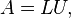LU Decomposition for solving linear equations in C++

Let A be a square matrix. An LU factorization refers to the factorization of A, with proper row and/or column orderings or permutations, into two factors, a lower triangular matrix L and an upper triangular matrix U,$A = LU, \,$
In the lower triangular matrix all elements above the diagonal are zero, in the upper triangular matrix, all the elements below the diagonal are zero. For example, for a 3-by-3 matrix A, its LU decomposition looks like this:$\begin{bmatrix} a_{11} & a_{12} & a_{13} \\ a_{21} & a_{22} & a_{23} \\ a_{31} & a_{32} & a_{33} \\ \end{bmatrix} = \begin{bmatrix} l_{11} & 0 & 0 \\ l_{21} & l_{22} & 0 \\ l_{31} & l_{32} & l_{33} \\ \end{bmatrix} \begin{bmatrix} u_{11} & u_{12} & u_{13} \\ 0 & u_{22} & u_{23} \\ 0 & 0 & u_{33} \\ \end{bmatrix}.$

Code for Program of Bresenham line drawing algorithm in C++ Programming

If we try out the C++ implementation of the Bresenham algorithm, we find it has some peculiar properties.
As expected, it fails to plot lines with negative slopes (try it and see what happens). It also fails to plot lines of positive slope greater than 1 (this is an interesting case, try it also and see if you can explain what is happening).
More unusually, we find that the order in which the endpoints are supplied to this routine is significant, it will only work as long as x1 is smaller than x2.
In fact, if we have two line segments with the same endpoints, and the same slope, this routine may draw one of them successfully but fails to draw the other one.

Transpose a matrix using c++ program

This c program prints transpose of a matrix. It is obtained by interchanging rows and columns of a matrix. For example if a matrix is
1 2
3 4
5 6
then transpose of above matrix will be
1 3 5
2 4 6
When we transpose a matrix then the order of matrix changes, but for a square matrix order remains same.

Difference of two matrices in c ++

An item in a matrix is called an entry or an element. The example has entries 1, 9, 13, 20, 55, and 6. Entries are often denoted by a variable with two subscripts. Thus in the matrix above, a2,1 = 20. Matrices of the same size can be added and subtracted entry-wise and matrices of compatible sizes can be multiplied. These operations have many of the properties of ordinary arithmetic, except that matrix multiplication is not commutative, that is, AB and BA are not equal in general.

Addition of two matrices using c++ program

This c program add two matrices i.e. compute the sum of two matrices and then print it. Firstly user will be asked to enter the order of matrix ( number of rows and columns ) and then two matrices. For example if the user entered order as 2, 2 i.e. two rows and two columns and matrices as

Matrix Multiplication in C++

Matrix multiplication in c language: c program to multiply matrices (two dimensional array), this program multiplies two matrices which will be entered by the user. Firstly user will enter the order of a matrix. If the entered orders of two matrix is such that they can't be multiplied then an error message is displayed on the screen. You have already studied the logic to multiply them in Mathematics. Matrices are frequently used while doing programming and are used to represent graph data structure, in solving system of linear equations and many more.

Upper-Triangular Matrix transform and solving Using Back-Substitution in c++

Definition (Upper-Triangular Matrix).

Anmatrixis called upper-triangular provided that the elements satisfywhenever.
If A is an upper-triangular matrix, thenis said to be an upper-triangular system of linear equations.